SAS Viya provides a robust, scalable, cloud-ready, distributed runtime engine. This engine is driven by CAS (Cloud Analytic Services), providing fast processing for many data management techniques that run distributive, i.e. using all threads on all defined compute nodes.

### Why

PROC APPEND is a common technique used in SAS processes. This technique will concatenate two data sets together. However, PROC APPEND will produce an ERROR if the target CAS table exists prior to the PROC APPEND.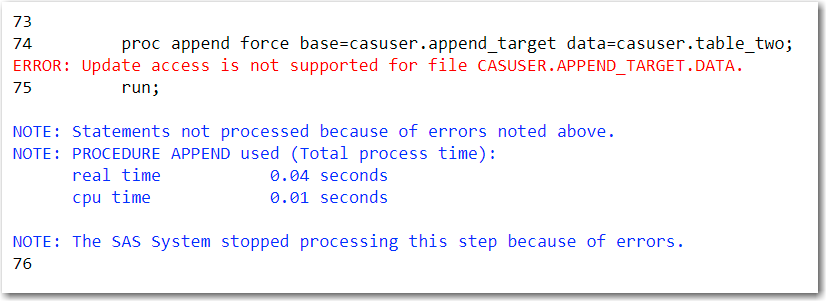Figure 1. SAS Log with the PROC APPEND ERROR message

Now what?

### How

To explain how to simulate PROC APPEND we first need to create two CAS tables. The first CAS table is named CASUSER.APPEND_TARGET. Notice the variables table, row and variable in figure 2.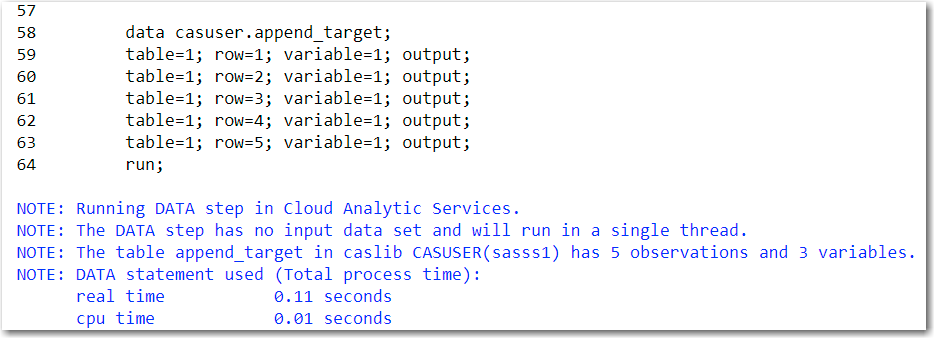Figure 2. Creating the CAS table we need to append rows to

The second CAS table is called CASUSER.TABLE_TWO and in figure 3 we can review the variables table, row, and variable.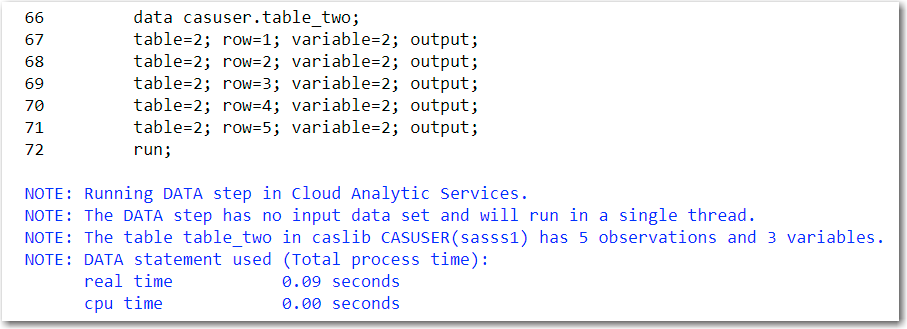Figure 3. Creating the table with the rows that we need to append to the existing CAS table

To simulate PROC APPEND we will use a DATA Step. Notice on line 77 in figure 4 we will overwrite an existing CAS table, i.e. CASUSER.APEND_TARGET. On Line 78, we see the first table on the SET statement is CASUSER.APPEND_TARGET, followed by CASUSER.TABLE_TWO. When this DATA Step runs, all of the rows in CASUSER.APPEND_TARGET will be processed first, followed by the rows in CASUSER.TABLE_TWO. Also, note we are not limited to two tables on the SET statement with DATA Step; we can use as many as we need to solve our business problems.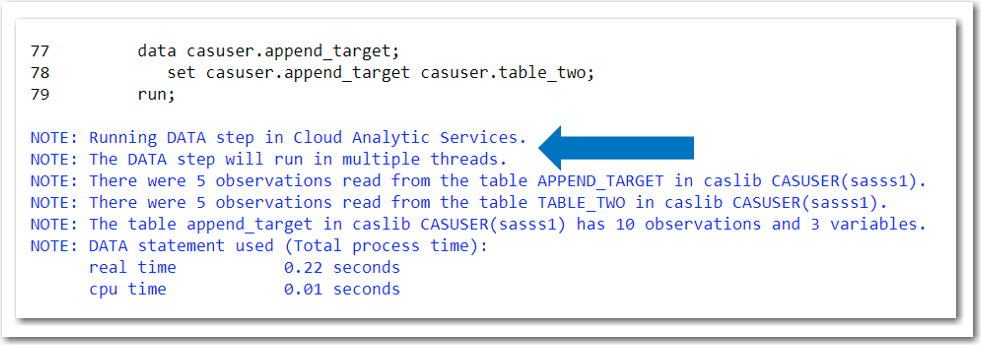Figure 4. SAS log validating the DATA Step ran in CAS i.e. distributed

The result table created by the DATA Step is shown in figure 5.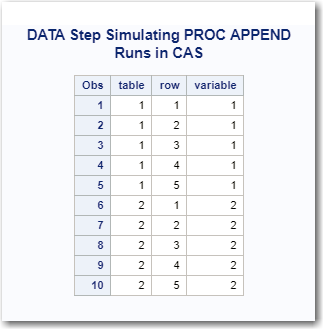Figure 5. Result table from simulating PROC APPEND using a DATA Step

### Conclusion

SAS Viya’s CAS processing allows us to stage data for downstream consumption by leveraging robust SAS programming techniques that run distributed, i.e. fast. PROC APPEND is a common procedure used in SAS processes. To simulate PROC APPEND when using CAS tables as source and target tables to the procedure use DATA Step.

How to simulate PROC APPEND in CAS was published on SAS Users.